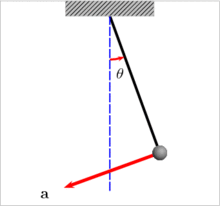## Centripetal Acceleration calculator

 I want to calculate Centripetal Acceleration(a) Radius(r) Velocity(v) Radius(r) = m Velocity(v) = m/s Centripetal Acceleration(a) = m/s2

Centripetal acceleration is the rate of change of tangential velocity.### Centripetal Acceleration formula:

Centripetal Acceleration: a=v2 / r

where v is Velocity and r means Circular Radius.

Thinkcalculator.com provides you helpful and handy calculator resources.